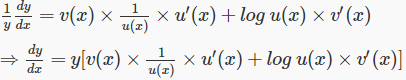# Logarithmic Differentiation

Logarithmic differentiation is a method to find the derivatives of some complicated functions, using logarithms. There are cases in which differentiating the logarithm of a given function is simpler as compared to differentiating the function itself. By the proper usage of properties of logarithms and chain rule finding, the derivatives become easy. This concept is applicable to nearly all the non-zero functions which are differentiable in nature.

Therefore, in calculus, the differentiation of some complex functions is done by taking logarithms and then the logarithmic derivative is utilized to solve such a function.

## Logarithmic Differentiation Formula

The equations which take the form y = f(x) = [u(x)]{v(x)} can be easily solved using the concept of logarithmic differentiation. The formula for log differentiation of a function is given by;

 d/dx(xx) = xx(1+ln x)

Get the complete list of differentiation formulas here.

For differentiating functions of this type we take on both the sides of the given equation.

Therefore, taking log on both sides we get,log y = log[u(x)]{v(x)}

log y = v(x)log u(x)

Now, differentiating both the sides w.r.t. x by implementing chain rule, we getThe only constraint for using logarithmic differentiation rules is that f(x) and u(x) must be positive as logarithmic functions are only defined for positive values.

The basic properties of real logarithms are generally applicable to the logarithmic derivatives.

For example: (log uv)’ = (log u + log v)’ = (log u)’ + (log v)’

### Method to Solve Logarithm Functions

Follow the steps given here to solve find the differentiation of logarithm functions.

• Find the natural log of the function first which is needed to be differentiated.
• Now by the means of properties of logarithmic functions, distribute the terms that were originally gathered together in the original function and were difficult to differentiate.
• Now differentiate the equation which was resulted.
• At last, multiply the available equation by the function itself to get the required derivative.

Now, as we are thorough with logarithmic differentiation rules let us take some logarithmic differentiation examples to know a little bit more about this.

### Problems

$$\begin{array}{l}\text{Q.1: Find the value of dy/dx if, }y = e^{x^{4}}\end{array}$$

Solution: Given function:

$$\begin{array}{l}y = e^{x^{4}}\end{array}$$

Taking natural logarithm of both the sides we get,

ln y = ln ex4

ln y = x4 ln e

ln y = x4

Now, differentiating both the sides w.r.t  we get,

$$\begin{array}{l}\frac{1}{y} \frac{dy}{dx} = 4x^3 \end{array}$$
$$\begin{array}{l} \Rightarrow \frac{dy}{dx}=y.4x^3\end{array}$$
$$\begin{array}{l}\Rightarrow \frac{dy}{dx}=e^{x^{4}}×4x^3\end{array}$$

Therefore, we see how easy and simple it becomes to differentiate a function using logarithmic differentiation rules.

$$\begin{array}{l}\text{Q.2: Find the value of }\frac{dy}{dx} \text{ if } y = 2x^{cos x}\end{array}$$

Solution: Given the function y = 2xcos x

Taking logarithm of both the sides, we get

log y = log(2x{cos x})

$$\begin{array}{l}\Rightarrow log y = log 2 + log x^{cos x} \\(As\ log(mn) = log m + log n)\end{array}$$

$$\begin{array}{l}\Rightarrow log y = log 2 + cos x × log x \\(As\ log m^n =n log m)\end{array}$$

Now, differentiating both the sides w.r.t by using the chain rule we get,

$$\begin{array}{l}\frac{1}{y} \frac{dy}{dx} = \frac{cos x}{x} – (sin x)(log x)\end{array}$$

This is yet another equation which becomes simplified after using logarithmic differentiation rules.

We have seen how useful it can be to use logarithms to simplify differentiation of various complex functions. Welcome to the world of BYJU’S to get to know more about differential calculus and also download the learning app.